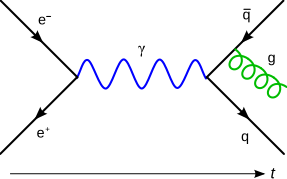# Feynman diagram facts for kids

Kids Encyclopedia FactsIn this Feynman diagram, an electron and positron destroy each other, producing a virtual photon which becomes a quark-antiquark pair. Then one radiates a gluon

A Feynman diagram is a diagram that shows what happens when elementary particles collide.

Feynman diagrams are used in quantum mechanics. A Feynman diagram has lines in different shapes—straight, dotted, and squiggly—which meet up at points called vertices. The vertices are where the lines begin and end. The points in Feynman diagrams where the lines meet represent two or more particles that happen to be at the same point in space at the same time. The lines in a Feynman diagram represent the probability amplitude for a particle to go from one place to another.

In Feynman diagrams, the particles are allowed to go both forward and backward in time. When a particle is going backward in time, it is called an antiparticle. The meeting points for the lines can also be interpreted forward or backwards in time, so that if a particle disappears into a meeting point, that means that the particle was either created or destroyed, depending on the direction in time that the particle came in from.

All the lines and vertices have an amplitude. When you multiply the probability amplitude for the lines, the amplitude for the particles to go from wherever they start to wherever they meet, and to the next meeting point, and so on, and also multiply by the amplitude for each meeting point, you get a number that tells you the total amplitude for the particles to do what the diagram says they do. If you add up all these probability amplitudes over all the possible meeting points, and over all the starting and ending points with an appropriate weight, you get the total probability amplitude for a collision in a particle accelerator, which tells you the total probability of these particles to bounce off one another in any particular direction.

Feynman diagrams are named after Richard Feynman, who won the Nobel Prize in Physics. His diagrams are very simple in the case of quantum electrodynamics (QED), where there are only two kinds of particles: electrons (little particles inside atoms) and photons (particles of light). In QED, the only thing that can happen is that an electron (or its antiparticle) can emit (or absorb) a photon, so there is only one building block for any collision. The probability amplitude for the emission is very simple—it has no real part, and the imaginary part is the charge of the electron.

## Images for kidsFeynman diagram Facts for Kids. Kiddle Encyclopedia.